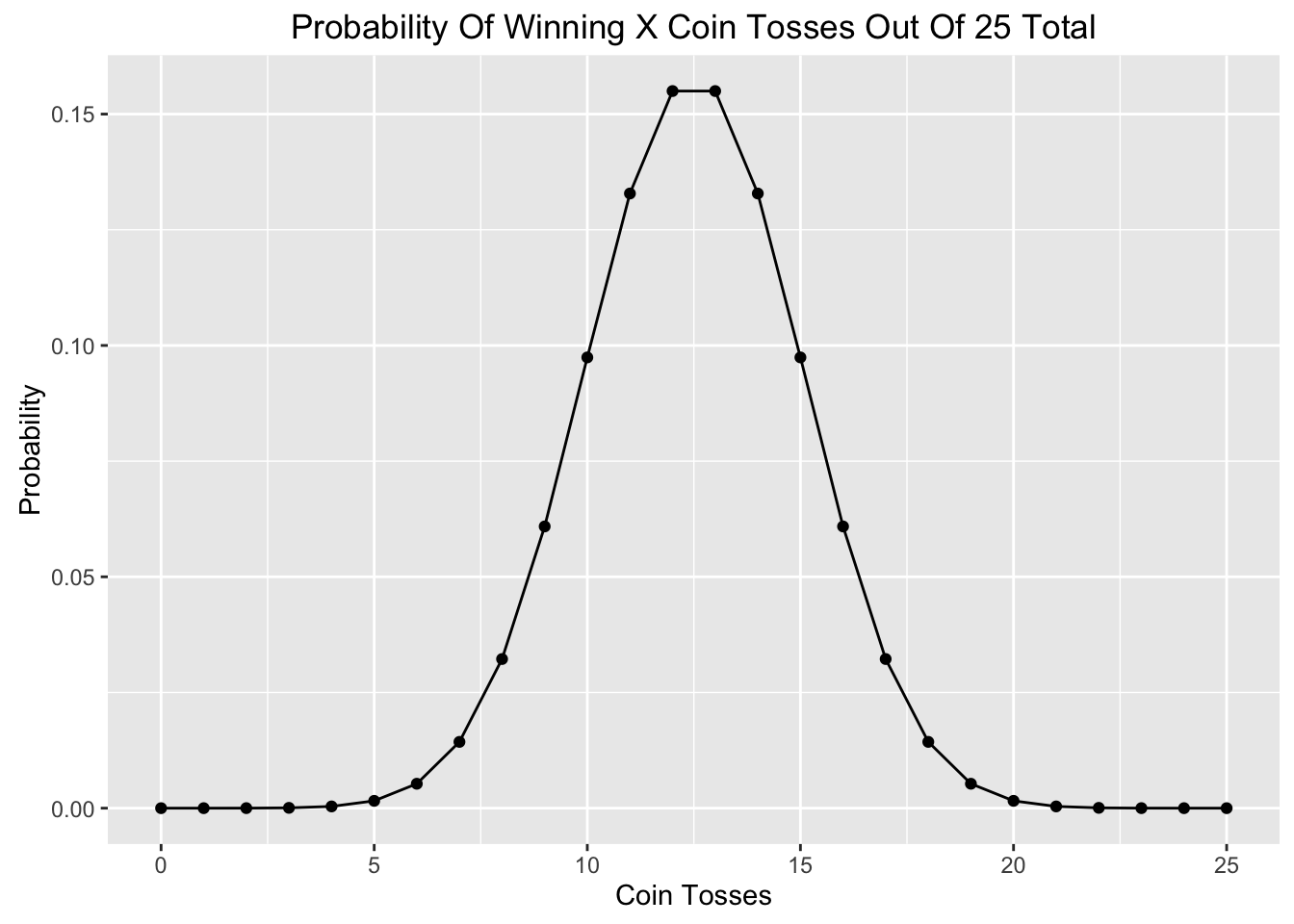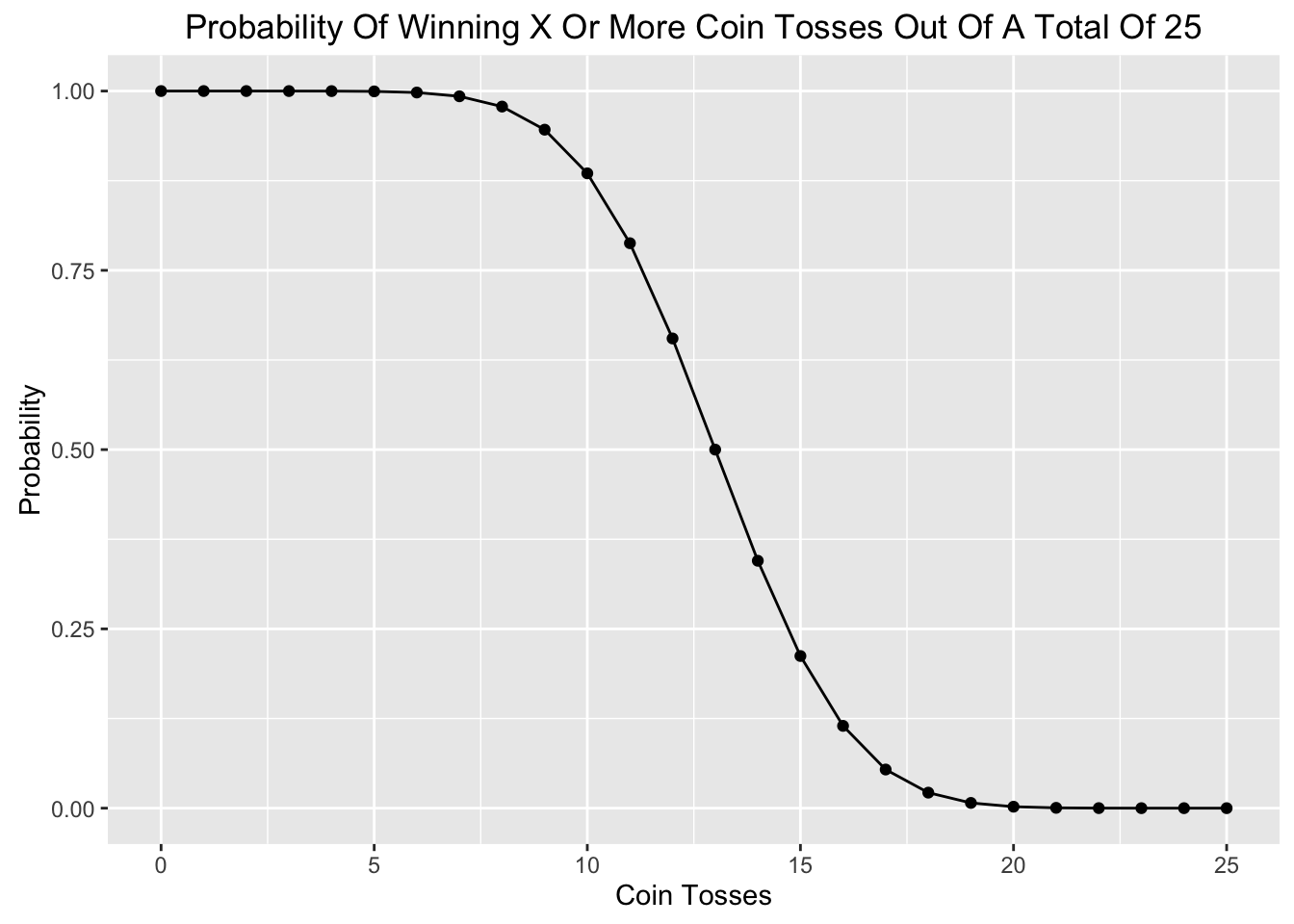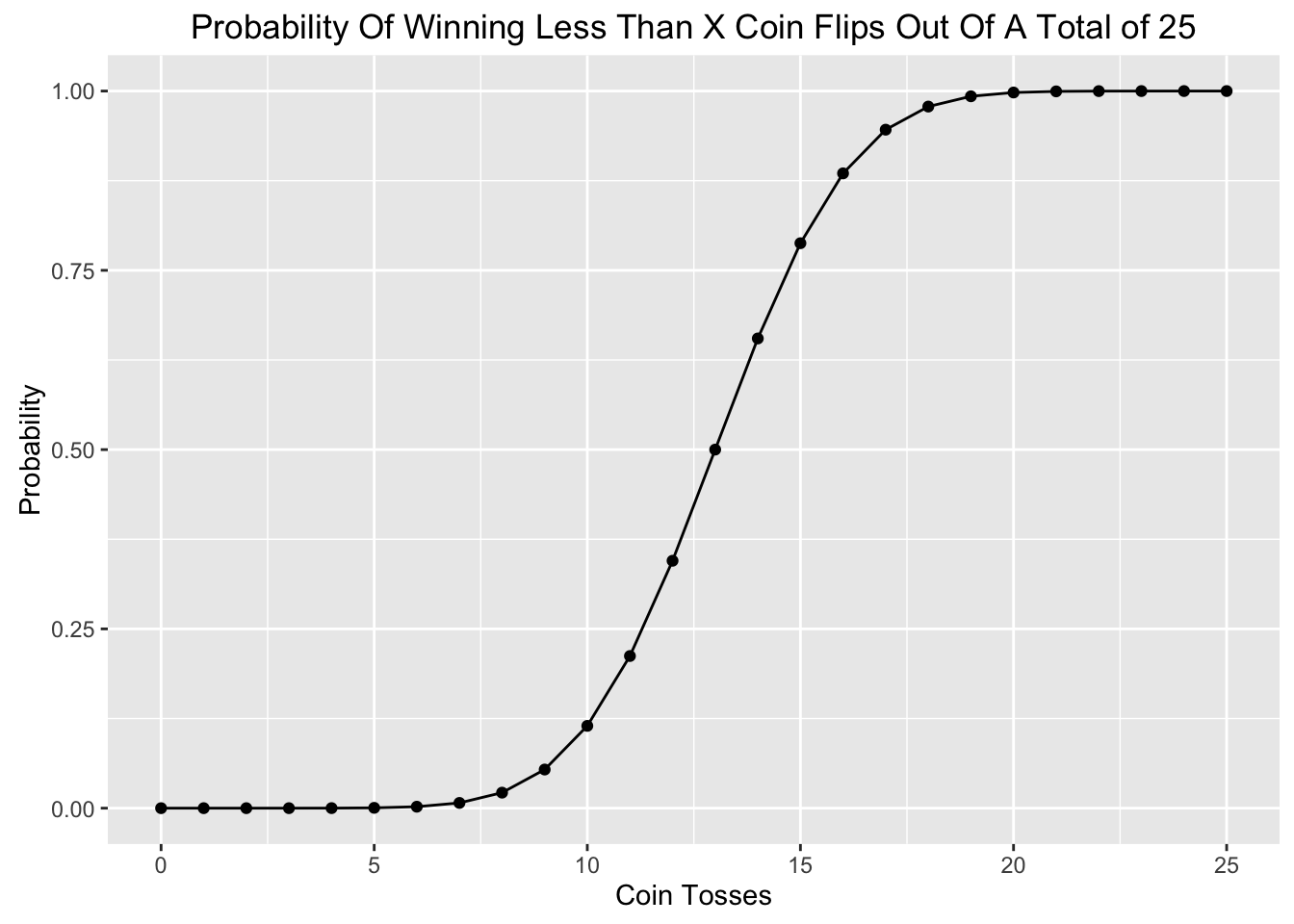Coin Toss Probability

##### What is the probability of the Patriots winning 19 out of 25 coin tosses.

According to this article as of November 25, 2015 the Patriots had won 19 out of the previous 25 coin tosses. Finding the probability of winning a series of coin tosses involves using the binomial distribution.

This is the formula for the binomial distribution:

$P(X)= \frac {n!} {(n-X)!X!} \cdot p^X \cdot q^{n-X}$

Where:

• n = number of trials
• X = number of successes in n trials
• p = probability of success
• q = probability of failure

The probability of this outcome looks like this:

$P(X)= \frac {25!} {(25-19)!19!} \cdot .5^{19} \cdot .5^{25-19} \approx 0.005277991294$

#### dbinom in R

Using R to find probability of winning exactly 19 out of 25 coin tosses is below:

probability <- .5
wins <- 19
totalFlips <- 25
dbinom(wins, totalFlips, probability)
##  0.005277991

So the probability of winning exactly 19 out of 25 coin flips is $$\approx$$.53% or approximately 1/2 of 1%.

#### Cumulative Binomial Probability

To find the probability of winning at least 19 flips in 25 trials we need to add the probability of getting between

$F(x) = P(X ≤ x)$

$F(x) = \sum\limits_{m=0}^x f(m) = f(0)+f(1)+ ... + f(x)$

The probability of winning at least 19 of 25 coin tosses is below. We find the probability of winning 18 or fewer coin tosses and then subract the result from 1 to get the probability of winning 19 or more times.

dbinom(0:18,25,0.5) # P of winning between 0 and 18 coin tosses
##   2.980232e-08 7.450581e-07 8.940697e-06 6.854534e-05 3.769994e-04
##   1.583397e-03 5.277991e-03 1.432598e-02 3.223345e-02 6.088540e-02
##  9.741664e-02 1.328409e-01 1.549810e-01 1.549810e-01 1.328409e-01
##  9.741664e-02 6.088540e-02 3.223345e-02 1.432598e-02
sum(dbinom(0:18,25,0.5)) # P of winning less than 19 times in 25 tries
##  0.9926834
1-sum(dbinom(0:18,25,0.5)) # P of winning more than 18 times in 25 tries.
##  0.007316649

#### pbinom

This same opeartion can be done using the pbinom function.

pbinom(wins-1, totalFlips, probability) # probability of winning 18 or fewer coin tosses
##  0.9926834

1-pbinom(wins-1, totalFlips, probability) # probability of winning 19 or more coin tosses
##  0.007316649

#### Plotting with ggplot2

Now we can plot the probability of winning different numbers of coin tosses. First we’ll create a data frame with three columns with the number of wins in one column, the probability of winning a specific number of coin tosses, and the probability of winning that number or more coin tosses.

library(ggplot2)

wins <- c(0:25)
totalFlips <- 25
probability <- .5

CoinFlips<- data.frame(wins=wins,probabilityXOrMoreWins = 1-pbinom(wins-1,totalFlips, probability), probabilityXWins=dbinom(wins,totalFlips,probability ))

tail(CoinFlips)
##   wins probabilityXOrMoreWins probabilityXWins
## 1    0              1.0000000     2.980232e-08
## 2    1              1.0000000     7.450581e-07
## 3    2              0.9999992     8.940697e-06
## 4    3              0.9999903     6.854534e-05
## 5    4              0.9999217     3.769994e-04
## 6    5              0.9995447     1.583397e-03
##    wins probabilityXOrMoreWins probabilityXWins
## 21   20           2.038658e-03     1.583397e-03
## 22   21           4.552603e-04     3.769994e-04
## 23   22           7.826090e-05     6.854534e-05
## 24   23           9.715557e-06     8.940697e-06
## 25   24           7.748604e-07     7.450581e-07
## 26   25           2.980232e-08     2.980232e-08
ggplot(CoinFlips, aes(wins, probabilityXWins)) + geom_point() + geom_line() + labs((list(title = "Probability Of Winning X Coin Tosses Out Of 25 Total", x = "Coin Tosses", y = "Probability")))ggplot(CoinFlips, aes(wins, probabilityXOrMoreWins)) + geom_point() + geom_line() + labs((list(title = "Probability Of Winning X Or More Coin Tosses Out Of A Total Of 25", x = "Coin Tosses", y = "Probability")))Now to find the probability of finding fewer than X wins in 25 trials.

CoinFlips<- cbind(CoinFlips, probabilityFewerThanXWins = pbinom(wins-1,totalFlips, probability))
head(CoinFlips)
##   wins probabilityXOrMoreWins probabilityXWins probabilityFewerThanXWins
## 1    0              1.0000000     2.980232e-08              0.000000e+00
## 2    1              1.0000000     7.450581e-07              2.980232e-08
## 3    2              0.9999992     8.940697e-06              7.748604e-07
## 4    3              0.9999903     6.854534e-05              9.715557e-06
## 5    4              0.9999217     3.769994e-04              7.826090e-05
## 6    5              0.9995447     1.583397e-03              4.552603e-04

And then plot.

ggplot(CoinFlips, aes(wins, probabilityFewerThanXWins)) + geom_point() + geom_line() + labs((list(title = "Probability Of Winning Less Than X Coin Flips Out Of A Total of 25", x = "Coin Tosses", y = "Probability")))